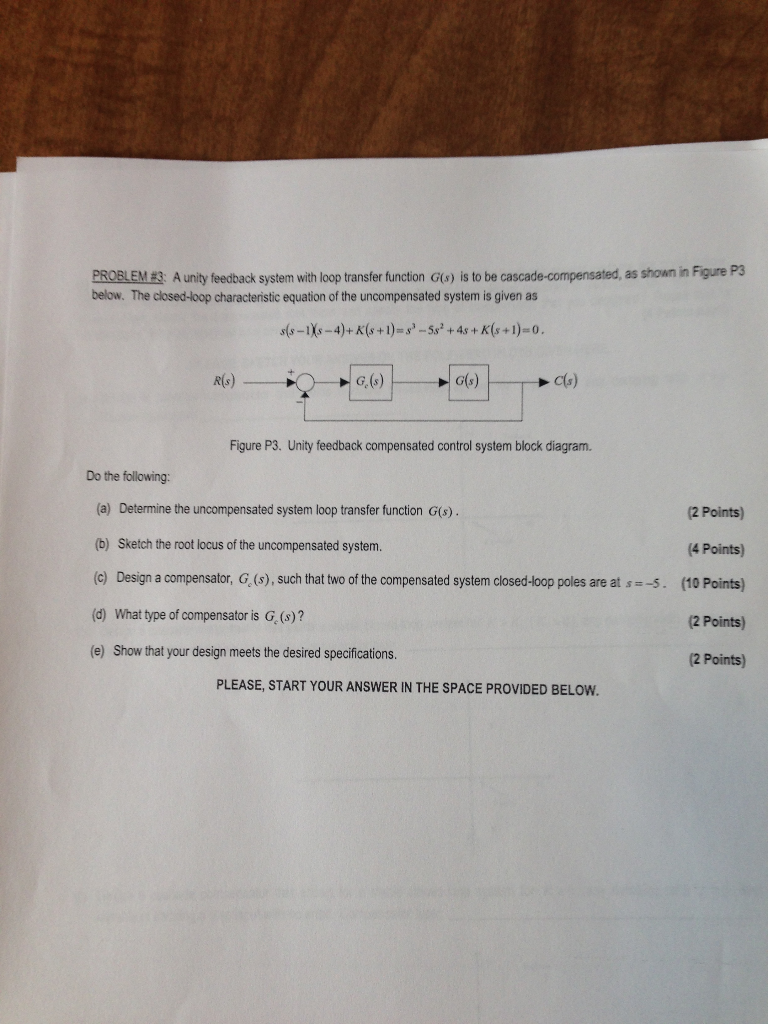# Stuck on this problem, an explanation of the answer would be very much appreciated!!

Stuck on this problem, an explanation of the answer would be very much appreciated!!PROBLEM E3 A unity feedback system with loop transfer function G(o) is to be cascade-compensated, as shown in Figure P3 below. The closed-loop characteristic equation of the uncompensated system is given as G. (s) G(s) Cs) Figure P3. Unity feedback compensated control system block diagram. Do the following: (a) Determine the uncompensated system loop transfer function G(s). b) Sketch the root locus of the uncompensated system. (c) Design a compensator, G, (s), such that two of the compensated system closed-loop poles are at s 5. (10 Points) d) What type of compensator is G. (s)? (e) Show that your design meets the desired specifications. 2 Points) (4 Points) 2 Points) 2 Points) PLEASE, START YOUR ANSWER IN THE SPACE PROVIDED BELOW##### Add Answer of: Stuck on this problem, an explanation of the answer would be very much appreciated!!
More Homework Help Questions Additional questions in this topic.

• #### photoelectric effect-your help would be very much appreciated

Need Online Homework Help?